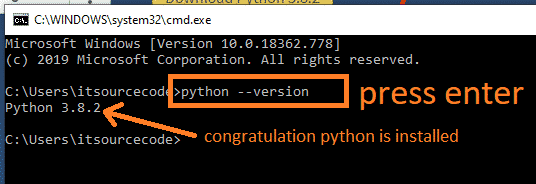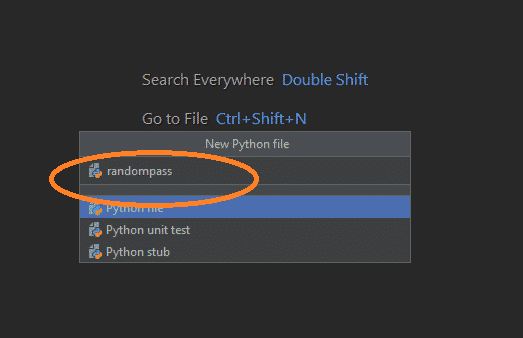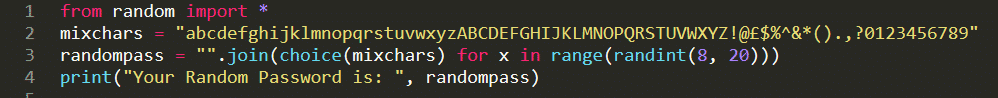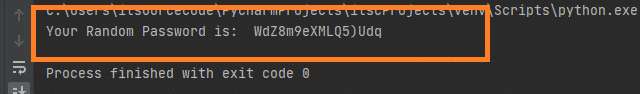# Random Password Generator in Python Projects With Source Code 2022

## Random Password Generator in Python Projects With Source Code 2022

In this tutorial, I will show you how Python Generate Random String and Password with Source code and Examples.

For the demonstration of this random password generator in python, I will use Python 3.8.2 version and Pycharm for my IDE.

By the way if you are new to python programming and you don’t know what would be the the Python IDE to use, I have here a list of Best Python IDE for Windows, Linux, Mac OS that will suit for you. I also have here How to Download and Install Latest Version of Python on Windows.

Before we proceed, watch the video tutorial here.

Python Generate Random String and Password

Go to official website of python and click the Download Python 3.8.2 button like as show below.2. Step 2: Install Python in your computer

3. Step 3: Test Python

To test python if it is successfully installed to your computer, open command prompt or press “Window + r” from your keyboard. then type cmd. When the command prompt open. Type “python –version”. see the image below.5. Step 5: Create new Project in Pycharm

Open Pycharm, Click File->New Project. Then specify the location where you store your project. In my case, I will use “itscProjects”. See the image below.6. Step 6: Create Python File

Right-click “Project”->Click “New”->Select “Python File”. See image below for reference.7. Step 7: Complete the creation of Python File

In the New Python File window, Type “randompass” and press enter.8. Step 8: Start coding

Note: I will add an embeddable code below so that you can copy and paste it to your editor.9. Step 9: Display Result from Generated Random Password in Python

when you execute the code in Step 8: you will have a result like as shown in the image.## Here’s the Code:

You can copy the code here and paste it in your code editor.

```from random import *
mixchars = "abcdefghijklmnopqrstuvwxyzABCDEFGHIJKLMNOPQRSTUVWXYZ!@£\$%^&*().,?0123456789"
randompass = "".join(choice(mixchars) for x in range(randint(8, 20)))

## Code Explanation

Let’s examine the code above.

In the first line of our code, we have random module which is use to provide access to functions that support many operations.

Second line, we use “mixchars” variable to hold some characters.

The random module in python is really useful especially when you want to create a secure password, it can allow you also to pick a random number in a given range.

Just like what we did in the third line of code. We use `randint()` functions of a random module to generate a random integer within a range.

and on the last line of code for random password generator, we display the Generated Random password.

## Upgrade Version of our Previous Codes

At this time we will explore more on another way of creating a random password generator in python. To start with, Let us try the following code first.

```import string
print(string.ascii_letters)
print(string.digits)
print(string.punctuation)```

And when you run the code above, it will give you a result looks like as show in the image below.

As you have notice, we have four(4) lines of code here. And I will explain each line.

Line 1: In this line, we use a python string module that contains a number of useful constants and classes which is useful in our python project to create a random password.

Line 2: In this line of code, we just simply display the list of ASCII letters both in a lower and upper case format.

Line 3: In this line of code, we just simply display the list of ASCII digits.

Line 4: In this line of code, we just simply display the list of ASCII punctuation.

At this time, we will now try to combine all of the codes from our previous version of the Random password generator in python. So here’s the following updated code.

```import string
from random import *
mixchars = string.ascii_letters+string.digits+string.punctuation
randompass = "".join(choice(mixchars) for x in range(randint(8, 20)))

You can observe in the code above that we have updated the value of “mixchars” variable from this

to this.

So what do you think? Which is better to use?. Please let me know by simply drop your comment below.

## Python Generate Random String

In this topic, we will create a python application to generate a random string characters.

```import random
import string

def randStr(strlen):
letters = string.ascii_letters
return ''.join(random.choice(letters) for i in range(strlen))

print("First Random String is ", randStr(8))
print("second Random String is ", randStr(8))```

What new to this code, is that we use function named “randStr” that this function will return a result later code.

Next, we also use “letters” variable to store the value from string asscii letters.

In the return, you will also notice that we use random.choice() to pick up a single character from the string constant.

Then, at the end of the iteration, it adds to the string variable using the join function.

Finally, we display the value from the function. See the image below.

## Python Generate Random String Without Repeating Characters

In our previous topic in python generate random string, we use there random.choice() to pick up a single character from the string constant.

But take note random.choice() might pick up same character again. So to eliminate this issue, we will use random.sample() function.

See the code below:

```import random
import string

def randStr(strlen=8):
letters = string.ascii_letters
return ''.join(random.sample(letters, strlen))

print("First Random String is ", randStr())
print("second Random String is ", randStr(8))```

the result of the code will be.

## Generate Random Password in Python that accepts user Input

At this time, we are going to generate random password in python that accept user inputs. For instance, when the program runs, it will ask the user to input how many number of password and the length of password to be generated. So let’s start.

For this demonstration, I will be using again random.choice function just like what I have discussed earlier.

```import string
import random

mxchars = string.ascii_letters+string.digits+string.punctuation

numInput = int(input("Enter the Number of passwords to Generate: "))
length = int(input("Enter the Password Length: "))
print("+++++++++++++++++++++++++++++++++++++++++++")
print("+++++++++++++++++++++++++++++++++++++++++++")
for pwd in range(numInput):
pw = ''
for c in range(length):
pw += random.choice(mxchars)
print(pw)```

And if you run the code, you will be asked to enter the number of password to be generated.

Then, when you press “Enter” you will be asked again to input the password length.

Finally, you will be asked to press “Enter” for the last time.

and you will be given a list of generated password like as shown below.

In this output, you will see that Python Generate Random String and Password with specific length.

This source code is is useful in creating a strong password that have mix of both lower and uppercase letters, numbers, and symbols. Again you can include this code in your main method. The passwords should be random, especially when generating new password every time the user asks for a new password.

## Final thought

In generating random password, there are so many ways and solution to do this. But again it’s up to you on how are your going to challenge yourself to dig deeper. But in our sample solution, we have made it to the point to make it clear, simple and elegant.

## What do you have in Mind?

I am excited to know your idea, what do you think in article? Is there anything I missed? I want you to know that I’m glad to hear from you. Please If you any questions or suggestions, please feel free to leave a comment below.